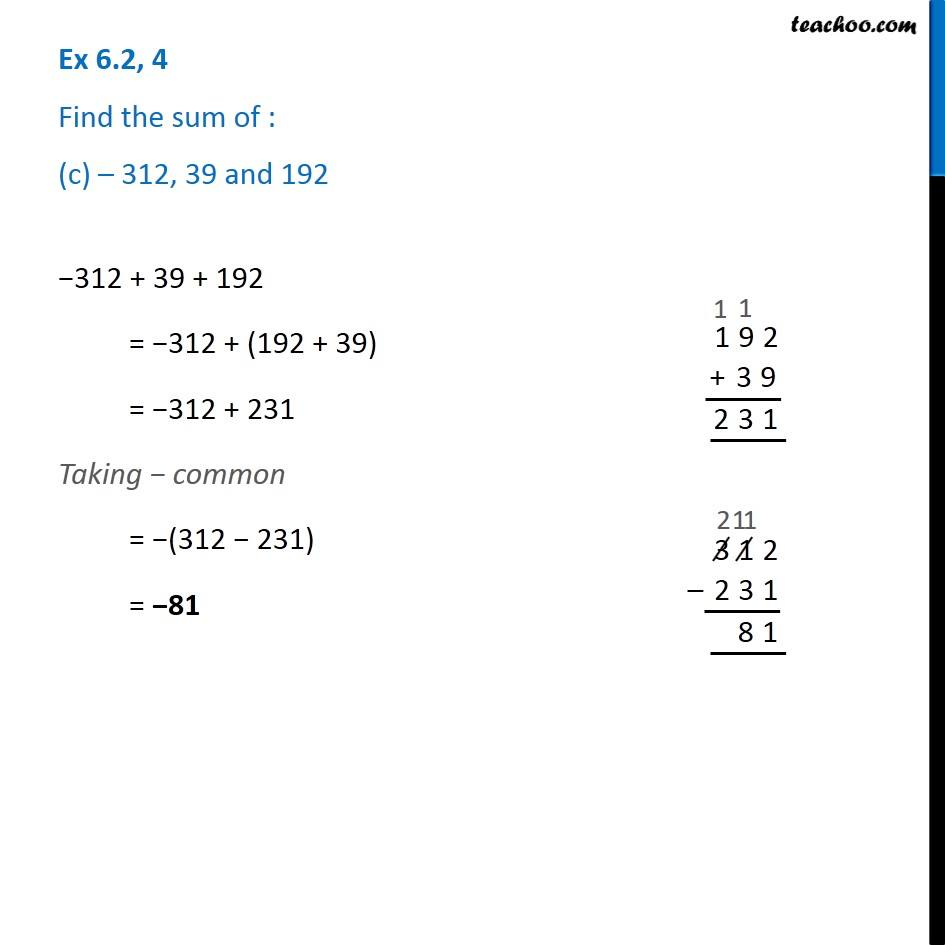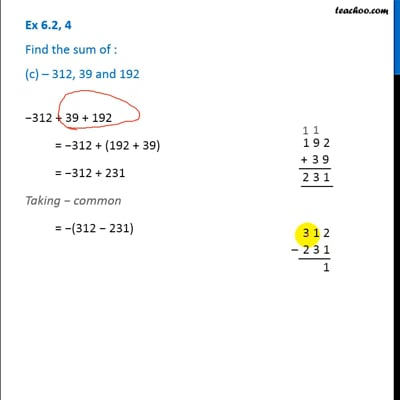Ex 6.2

Chapter 6 Class 6 Integers
Serial order wiseThis video is only available for Teachoo black users

### Transcript

Ex 6.2, 4 Find the sum of : (c) – 312, 39 and 192 −312 + 39 + 192 = −312 + (192 + 39) = −312 + 231 Taking − common = −(312 − 231) = −81